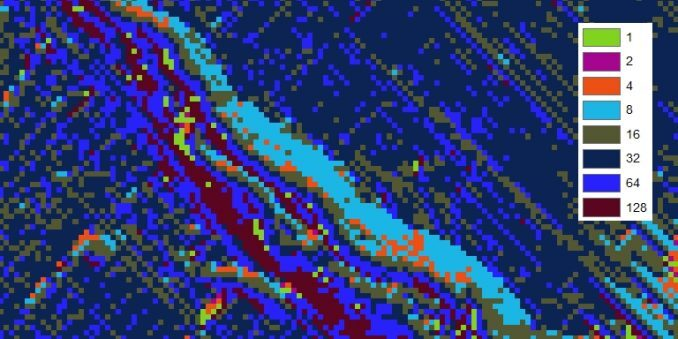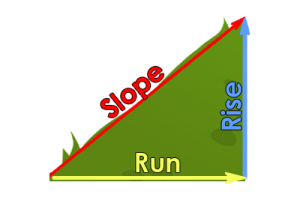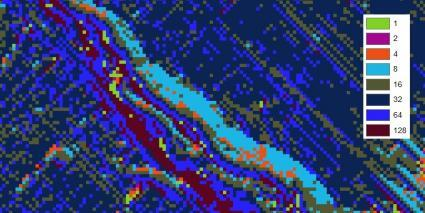Home » GIS Analysis » Flow Direction Calculates Water Direction Using Slope

# Flow Direction Calculates Water Direction Using Slope### What is flow direction?

Hydrologists use flow direction maps to help model how surface runoff contributes to flooding. They also use it for a lot more.

Flow direction calculates the direction water will flow using slope from neighboring cells.

But how does flow direction really work?

Today, we explore the hydrology tool of flow direction. On a cell-by-cell basis, we explore which direction water travels using a pour-point model.

### How to calculate flow direction

First, start off with a digital elevation model like from one of these free DEM data sources. Flow direction determines which direction water will flow in a given cell.

Based on the direction of the steepest descent in each cell, we measure flow direction. Also, the z-value difference and slope are calculated between neighboring cells.In a given grid cell, water can flow to one or more of its eight adjacent cells. The slope is the ultimate factor in how water flows in this model.

We use a pour-point model to show how and in which direction water travels. The eight adjacent cells in the pour point model has a value expressing how water drops.

### Flow direction example

When water flows in the east direction, it has a value of 1. When water flows west, it has a value of 16. All 8 adjacent directions at a given point can be described using the eight-direction pour point model.When running the flow direction algorithm, the resulting values range from 1, 2, 4, 8, 16, 32, 64, and 128. In the eight direction pour-point model diagram, you can understand which flow direction water travels.